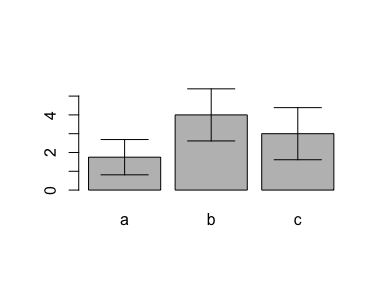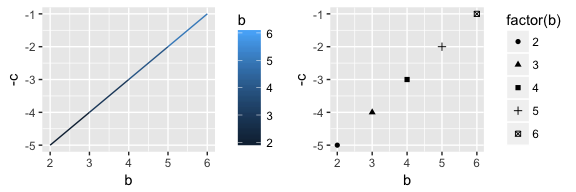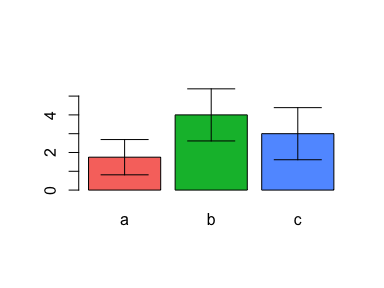Package miscset introduction

2017-02-24

R package miscset version 1.1.0.

A collection of miscellaneous methods to simplify various tasks, including plotting, data.frame and matrix transformations, environment functions, regular expression methods, and string and logical operations, as well as numerical and statistical tools.

Most of the methods are simple but useful wrappers of common base R functions, which extend S3 generics or provide default values for important parameters.

Installation and Introduction

install.packages('miscset')

Install the development version from github via:

install.packages('devtools')
devtools::install_github('setempler/miscset@develop', build_vignettes = TRUE)

After installation, load the package via

library(miscset)

If you like to contribute to the development of the packages, please

• find the source code at github
• contribute with filing an issues

Get help in an R session via

• help.index(miscset)
• ? + function name

Plot methods

Function ciplot

Plot a bargraph with error bars. Input data is a list with numeric vectors. Functions to calculate bar heights (e.g. mean by default) and error bar sizes (e.g. confint.numeric by default) can be modified (e.g. sd for error bars).

d <- data.frame(a=c(2,1,3,NA,1), b=2:6, c=5:1)
ciplot(d)Function ggplotGrid

Arrange ggplots on a grid (plot window or pdf file). Supply a list with ggplot objects and define number of rows and/or columns. If a path is supplied, the plot is written to that file instead of the internal graphics device.

library(ggplot2)
plots <- list(
ggplot(d, aes(x = b, y= -c, col = b)) + geom_line(),
ggplot(d, aes(x = b, y = -c, shape = factor(b))) + geom_point())
ggplotGrid(plots, ncol = 2)The function ggplotGridA4 supports direct output to DIN A4 sized pdfs.

Function gghcl

Generate a character vector with html values from a color hue as in ggplot.

d <- data.frame(a=c(2,1,3,NA,1), b=2:6, c=5:1)
n <- length(d)
gghcl(n)
 "#F8766D" "#00BA38" "#619CFF"
ciplot(d, col = gghcl(n))Function plotn

Create an empty plot. Useful to fill layout.

plotn()Data Frame and Matrix Methods

Function sort

Sort data.frame objects. This extends the functionality of the base R distributed generic sort. Define multiple columns by column names as character vector or expression.

d <- data.frame(a=c(2,1,3,NA,1), b=2:6, c=5:1)
print(d)
a b c
1  2 2 5
2  1 3 4
3  3 4 3
4 NA 5 2
5  1 6 1
sort(d, by = c("a", "c"))
a b c
5 1 6 1
2 1 3 4
1 2 2 5
3 3 4 3

Function do.rbind

Note: This function is now deprecated. It is recommended to use rbindlist from the data.table package.

A wrapper function to row-bind data.frame objects in a list with do.call and rbind. Object names from the list are inserted as additional column.

d <- data.frame(a=c(2,1,3,NA,1), b=2:6, c=5:1)
print(d[1:3,])
a b c
1 2 2 5
2 1 3 4
3 3 4 3
do.rbind(list(first=d[1:2,], second=d[1:3,]))
Warning: 'do.rbind' is deprecated.
See help("Deprecated")
Name a b c
1  first 2 2 5
2  first 1 3 4
3 second 2 2 5
4 second 1 3 4
5 second 3 4 3

Function enpaire

Generate a pairwise list (data.frame) of a matrix containing row and column id and upper and lower triangle values.

m <- matrix(letters[1:9], 3, 3, dimnames = list(1:3,1:3))
print(m)
1   2   3
1 "a" "d" "g"
2 "b" "e" "h"
3 "c" "f" "i"
enpaire(m)
row col lower upper
1   1   2     b     d
2   1   3     c     g
3   2   3     f     h

Function squarematrix

Generate a symmetric (square) matrix from an unsymmetric one using column and row names. Fills empty cells with NA.

m <- matrix(letters[1:9], 3, 3, dimnames = list(1:3,1:3))
print(m[-1,])
1   2   3
2 "b" "e" "h"
3 "c" "f" "i"
squarematrix(m[-1,])
1   2   3
1 NA  NA  NA
2 "b" "e" "h"
3 "c" "f" "i"

Function textable

Print a data.frame as latex table. Extends xtable by optionally including a latex header, and if desired writing the output to a file directly and calling a system command to convert it to a .pdf file, for example.

d <- data.frame(a=c(2,1,3,NA,1), b=2:6, c=5:1)
textable(d, caption = 'miscset vignette example data.frame', as.document = TRUE)
% output by function 'textable' from package miscset 1.1.0
% latex table generated in R 3.3.2 by xtable 1.8-2 package
% Fri Feb 24 02:59:22 2017

\documentclass[a4paper,10pt]{article}
\usepackage[a4paper,margin=2cm]{geometry}
\begin{document}

\begin{table}[ht]
\centering
\caption{miscset vignette example data.frame}
\begin{tabular}{rrr}
\hline
a & b & c \\
\hline
2.00 &   2 &   5 \\
1.00 &   3 &   4 \\
3.00 &   4 &   3 \\
&   5 &   2 \\
1.00 &   6 &   1 \\
\hline
\end{tabular}
\end{table}

\end{document}

Environment Functions

Function help.index

Show the help index page of a package (with the list of all help pages of a package).

help.index(miscset)

Load multiple R data objects into a list. List is of same length as number of files provided. Sublists contain all respective objects. Simplification is possible if all names are unique.

Function lsall

Return all current workspace (or any custom) object names, lengths, classes, modes and sizes in a data.frame.

lsall()
Environment: R_GlobalEnv
Objects:
Name Length      Class      Mode   Size Unit
1     d      3 data.frame      list 1008.0 byte
2     m      9     matrix character    1.3   Kb
3     n      1    integer   numeric   48.0 byte
4 plots      2       list      list   10.9   Kb

Function rmall

Remove all objects from the current or custom environment.

rmall()

Regular Expression Methods

Function mgrepl

Search for multiple patterns in a character vector. Merge results by (custom) logical functions (e.g. any, all) and use mutlicore support from the parallel package. Optionally return the index (as with which). Use identity to return a matrix with the results of each pattern per row.

s <- c("ab","ac","bc", NA)
mgrepl(c("a","b"), s)
  TRUE FALSE FALSE FALSE
mgrepl(c("a","b"), s, any) # similar to: grepl("a|b", s)
  TRUE  TRUE  TRUE FALSE
mgrepl(c("a", "b"), s, sum)
 2 1 1 0
mgrepl(c("a","b"), s, identity)
[,1]  [,2]  [,3]  [,4]
[1,] TRUE  TRUE FALSE FALSE
[2,] TRUE FALSE  TRUE FALSE

Function gregexprind

Retreive the nth or "last" index of an expression found in a character string.

gregexprind(c("a"), c("ababa","ab","xyz",NA), 1)
  1  1 NA NA
gregexprind(c("a"), c("ababa","ab","xyz",NA), 2)
  3 NA NA NA
gregexprind(c("a"), c("ababa","ab","xyz",NA), "last")
  5  1 NA NA

String and Logical Methods

Function collapse

To collapse vectors, usually a call to paste or paste0 setting the argument collapse is applied. The collapse function is a wrapper of this functionality applied to a single vector. It can be extended with the .unique, .sort and .decreasing arguments, to return only unique and sorted values.

paste(letters, collapse = "")
 "abcdefghijklmnopqrstuvwxyz"
collapse(letters)
 "abcdefghijklmnopqrstuvwxyz"

The data.frame method allows to collapse a data frame by identifier/grouping columns (specified with by). Each group piece has then all value columns collapsed with the default method.

In addition, the value columns can be collapsed to vectors, when sep = NULL is selected, keeping a list of vectors for this column in the returned data frame. .sortby allows to choose if the result should be sorted by the grouping columns. .unlist provides a way to unlist value columns per group, which is useful if the input has list columns.

# create example data
set.seed(12)
s <- s2 <- sample(LETTERS[1:4], 9, replace = TRUE)
s2[1:2] <- rev(s2[1:2])
d <- data.frame(group = rep(letters[c(3,1,2)], each = 3),
value = s,
level = factor(s2),
stringsAsFactors = FALSE)
print(d)
group value level
1     c     A     D
2     c     D     A
3     c     D     D
4     a     B     B
5     a     A     A
6     a     A     A
7     b     A     A
8     b     C     C
9     b     A     A

The following (default settings) collapses by all columns, which results in an output similar to unique(d), but the row names are not kept.

collapse(d)
group value level
1     c     A     D
2     c     D     A
3     c     D     D
4     a     B     B
5     a     A     A
6     b     A     A
7     b     C     C

Specifying no grouping columns (setting by to 0 or NULL) collapses all columns.

collapse(d, by = NULL)
group     value     level

Specifying at least one and maximum less than the total columns groups the data.frame, splits it into group pieces, and applies the collapsing to all remaining columns.

collapse(d, "/", 1)
group value level
1     c A/D/D D/A/D
2     a B/A/A B/A/A
3     b A/C/A A/C/A

If the separator sep is not specified, the data.frame method allows to return list columns, containing vectors of values per group. With the .sortby argument, the ouptut can be sorted on the grouping values.

# by first column, but keep values as vectors
collapse(d, NULL, c(1,3), .sortby = T)
group level value
1     a     A  A, A
2     a     B     B
3     b     A  A, A
4     b     C     C
5     c     A     D
6     c     D  A, D

The data.frame method also works on data.table objects, since it uses the methods from the package of the same name to split the input into group pieces. If the input inherits from data.table, the class is retained.

Prepend 0 characters to numbers to generate equally sized strings.

 "0009" "0112" "5009"

Function strextr

Note: This function is now deprecated. It is recommended to use str_extract or str_extract_all from the stringr package.

Split strings by a separator (sep) and extract all substrings matching a pattern. Optionally allow multiple matches, and use multicore support from the parallel package.

s <- "xa,xb,xn,ya,yb"
strextr(s, "n$", ",") Warning: 'strextr' is deprecated and will be removed with the release of miscset version 2. Use 'stringr::str_extract' instead. See examples in ?strextr  "xn" strextr(s, "^x", ",", mult=T) Warning: 'strextr' is deprecated and will be removed with the release of miscset version 2. Use 'stringr::str_extract' instead. See examples in ?strextr []  "xa" "xb" "xn" library(stringr) str_extract(s, "[^,]*n")  "xn" str_extract_all(s, "x[^,]*") []  "xa" "xb" "xn" Function str_part Similar to strextr, but extracting substrings is done by setting an index value n. Optionally roll the last value to n if it’s index is less. s <- "xa,xb,xn,ya,yb" str_part(s, ",", 3)  "xn" Function str_rev Create reverse version of strings of a character vector. str_rev(c("olleH", "!dlroW"))  "Hello" "World!" Function duplicates and duplicatei Determine duplicates. Return either a logical vector (duplicates) or an integer index (duplicatei). Extends the base method duplicated by also returning TRUE for the first occurence of a value. data.frame( duplicate = d$a,
".d" = duplicated(d$a), # standard R function ".s" = duplicates(d$a),
".i" = duplicatei(d\$a))
 .d .s .i
<0 rows> (or 0-length row.names)

Numeric Methods

Function p2star

Asign range symbols to values, e.g. convert p-values to significance characters.

p2star(c(0.003, 0.049, 0.092, 0.431))
 "**"   "*"    "."    "n.s."

Function confint.numeric

Calculate confidence intervals. Extends the base method confint to numeric vectors.

n <- c(2,1,3,NA,1)
confint(n, ret.attr = FALSE)
 0.8392064

Function ntri

Generate a series of triangular numbers of length n according to OEIS#A000217. The series for 12 rows of a triangle, for example, can be returned as in the following example.

ntri(12)
  0  1  3  6 10 15 21 28 36 45 55 66

Function scale0 and scaler

Scale numeric vectors to a range of 0 to 1 with scale0 or to a custom output range r and input range b with scaler.

n <- 5:1
scale0(n)
 1.00 0.75 0.50 0.25 0.00
scaler(n, c(2, 6), b = c(1, 10))
 3.777778 3.333333 2.888889 2.444444 2.000000

Function nunique and uniquei

Return the amount (with nunique) or index (with uniquei) of unique values in a vector. Extends plyr::nunique by allowing NA values to be counted as a ‘level’.

n <- c(2,1,3,NA,1)
nunique(n)
 4
nunique(n, FALSE)
 3
uniquei(n)
 1 2 3 4
uniquei(n, FALSE)
 1 2 3